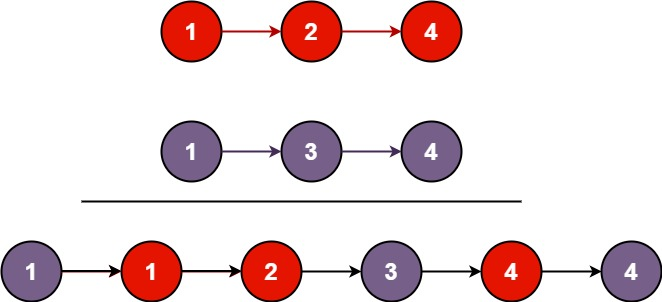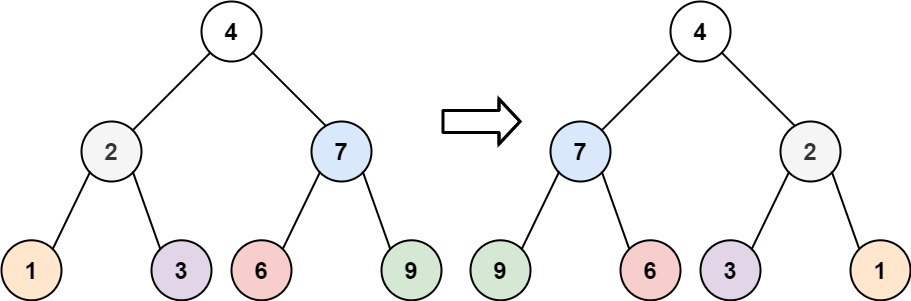# Week 1 | Grind 169 | Blind 75

Posted on Dec 25, 2020

## 1. Two Sum

Given an array of integers nums and an integer `target`, return indices of the two numbers such that they add up to `target`.

You may assume that each input would have exactly one solution, and you may not use the same element twice.

You can return the answer in any order.

``````vector<int> twoSum(vector<int>& nums, int target) {
unordered_map<int, int> ump;
int check, f(-1), s(-1);
for(int i=0; i<nums.size(); i++){
check = target - nums[i];
if(ump.find(check) != ump.end()) {
f = ump[check];
s = i;
break;
}else ump[nums[i]] = i;
}
return {f, s};
}
``````

## 2. Valid Parentheses

Given a string s containing just the characters ‘(’, ‘)’, ‘{’, ‘}’, ‘[’ and ‘]’, determine if the input string is valid.

An input string is valid if:

• Open brackets must be closed by the same type of brackets.

• Open brackets must be closed in the correct order.

• Practice

``````bool isValid(string s) {
stack<char> st;
for(char c : s){
if(c == '('|| c == '{' || c == '['){
st.push(c);
}else{
if(st.empty()) return false;
if(c == ')' && st.top() != '(') return false;
if(c == '}' && st.top() != '{') return false;
if(c == ']' && st.top() != '[') return false;
st.pop();
}
}
return st.empty();
}
``````

## 3. Merge Two Sorted Lists

You are given the heads of two sorted linked lists list1 and list2.

Merge the two lists in a one sorted list. The list should be made by splicing together the nodes of the first two lists.``````ListNode* mergeTwoLists(ListNode* l1, ListNode* l2) {

while (l1 && l2) {
if (l1->val < l2->val) {
tail->next = l1;
l1 = l1->next;
} else {
tail->next = l2;
l2 = l2->next;
}
tail = tail->next;
}

tail->next = l1 ? l1 : l2;

}
``````

## 4. Best Time to Buy and Sell Stock

You are given an array `prices` where `prices[i]` is the price of a given stock on the ith day.

You want to maximize your profit by choosing a single day to buy one stock and choosing a different day in the future to sell that stock.

Return the maximum profit you can achieve from this transaction. If you cannot achieve any profit, return 0.

``````int maxProfit(vector<int>& prices) {
int buy = INT_MAX, profit = 0;
for (auto price: prices){
}
return profit;
}
``````

## 5. Valid Palindrome

A phrase is a palindrome if, after converting all uppercase letters into lowercase letters and removing all non-alphanumeric characters, it reads the same forward and backward. Alphanumeric characters include letters and numbers.

Given a string s, return true if it is a palindrome, or false otherwise.

``````bool isPalindrome(string s) {
string pal;

if(!s.size()) return true;

for(const auto &ch: s){
if(isalnum(ch)){
if(isupper(ch)) pal += ch+32;
else pal += ch;
}
}

int n = pal.size();

for(int i=0; i<n/2; i++)
if(pal[i] != pal[n-i-1])
return false;

return true;
}
``````

## 6. Invert Binary Tree

Given the root of a binary tree, invert the tree, and return its root.### DFS

``````    TreeNode* invertTree(TreeNode* root) {

if(!root) return NULL;

TreeNode* tmp = invertTree(root->right);
root->right = invertTree(root->left);
root->left = tmp;

return root;
}
``````

### BFS

``````    TreeNode* invertTree(TreeNode* root) {
stack<TreeNode*> st;
st.push(root);

while (!st.empty()) {
TreeNode* curr = st.top();
st.pop();
if (!curr) continue;
st.push(curr->left);
st.push(curr->right);
swap(curr->left, curr->right);
}
return root;
}
``````

## 7. Valid Anagram

Given two strings `s` and `t`, return true if t is an anagram of s, and false otherwise.

An `Anagram` is a word or phrase formed by rearranging the letters of a different word or phrase, typically using all the original letters exactly once.

``````bool isAnagram(string s, string t) {
int hash = {0};

if(s.length() != t.length())
return false;

for(char ch:s)
hash[ch-97]++;

for(char ch:t)
if(hash[ch-97])
hash[ch-97]--;
else
return false;

return true;
}
``````

Given an array of integers nums which is sorted in ascending order, and an integer `target`, write a function to search target in nums. If `target` exists, then return its index. Otherwise, return -1.

You must write an algorithm with `O(log n)` runtime complexity.

``````int search(vector<int>& nums, int target) {
int mid, l = 0, r = nums.size() - 1;
while(l<=r){
mid = l + (r-l)/2;
if(nums[mid] == target) return mid;
if(nums[mid] < target)
l = mid+1;
else
r = mid-1;
}
return -1;
}
``````

## 10. Maximum Subarray

Given an integer array `nums`, find the contiguous subarray (containing at least one number) which has the largest sum and return its sum.

A subarray is a contiguous part of an array.

``````int maxSubArray(vector<int>& nums) {

int maxi = INT_MIN, sum = 0, n = nums.size();

for(const auto &e: nums){
sum += e;
maxi = max(maxi, sum);
if(sum < 0) sum  = 0;
}

return maxi;
}
``````

## 11. Lowest Common Ancestor of a Binary Search Tree

Given a binary search tree (BST), find the lowest common ancestor (LCA) of two given nodes in the BST.

According to the `definition of LCA on Wikipedia:` “The lowest common ancestor is defined between two nodes p and q as the lowest node in T that has both p and q as descendants (where we allow a node to be a descendant of itself).”

``````TreeNode* lowestCommonAncestor(TreeNode* root, TreeNode* p, TreeNode* q) {

if(p->val < root->val && q->val < root->val)
return lowestCommonAncestor(root->left,p,q);

if(p->val > root->val && q->val > root->val)
return lowestCommonAncestor(root->right,p,q);

return root;
}
``````

## 12. Balanced Binary Tree

Given a binary tree, determine if it is height-balanced.

For this problem, a height-balanced binary tree is defined as:

A binary tree in which the left and right subtrees of every node differ in height by no more than 1.

``````int height(TreeNode* root) {
if (!root) return 0;
int left = height(root->left);
int right = height(root->right);
return (left == -1 || right == -1 || abs(left-right) > 1) ? -1 : max(left, right) + 1;
}

bool isBalanced(TreeNode* root) {
return height(root) != -1;
}
``````

Given `head`, the head of a linked list, determine if the linked list has a cycle in it.

There is a cycle in a linked list if there is some node in the list that can be reached again by continuously following the `next` pointer. Internally, pos is used to denote the index of the node that tail’s `next` pointer is connected to. Note that pos is not passed as a parameter.

Return `true` if there is a cycle in the linked list. Otherwise, return `false`.

``````bool hasCycle(ListNode *head) {

while(fast && fast->next){
slow=slow->next;
fast=fast->next->next;
if(fast==slow) return true;
}
return false;
}
``````

## 14. Implement Queue using Stacks

Implement a first in first out (FIFO) queue using only two stacks. The implemented queue should support all the functions of a normal queue (push, peek, pop, and empty).

Implement the `MyQueue` class:

• void push(int x) Pushes element x to the back of the queue.
• int pop() Removes the element from the front of the queue and returns it.
• int peek() Returns the element at the front of the queue.
• boolean empty() Returns true if the queue is empty, false otherwise.

Notes:

• You must use only standard operations of a stack, which means only push to top, peek/pop from top, size, and is empty operations are valid.

• Depending on your language, the stack may not be supported natively. You may simulate a stack using a list or deque (double-ended queue) as long as you use only a stack’s standard operations.

• Practice

### O(1) push

``````class MyQueue {
public:
stack<int> que;
stack<int> tmp;
int e;
MyQueue() {
}

void push(int x) {

while(!que.empty()){
tmp.push(que.top());
que.pop();
}

tmp.push(x);

while(!tmp.empty()){
que.push(tmp.top());
tmp.pop();
}
}

int pop() {
e = que.top();
que.pop();
return e;
}

int peek() {
return que.top();
}

bool empty() {
return que.empty();
}
};
``````

### O(1) push

``````class MyQueue {
public:
stack<int> que;
stack<int> tmp;
int front;
MyQueue() {
}

void push(int x) {
que.push(x);
}

int pop() {

while(!que.empty()){
tmp.push(que.top());
que.pop();
}

front = tmp.top();
tmp.pop();

while(!tmp.empty()){
que.push(tmp.top());
tmp.pop();
}
return front;
}

int peek() {

while(!que.empty()){
tmp.push(que.top());
que.pop();
}

front = tmp.top();

while(!tmp.empty()){
que.push(tmp.top());
tmp.pop();
}
return front;
}

bool empty() {
return que.empty();
}
};
``````

### Amortized O(1) Push and Pop

``````class MyQueue {
public:
stack<int> in;
stack<int> out;
int front;
MyQueue() {
}

void push(int x) {
in.push(x);
}

int pop() {

if(out.empty()) {
while(!in.empty()) {
out.push(in.top());
in.pop();
}
}

front = out.top();
out.pop();
return val;
}

int peek() {

if(out.empty()) {
while(!in.empty()) {
out.push(in.top());
in.pop();
}

}

return out.top();
}

bool empty() {
return in.empty();
}
};
``````

You are a product manager and currently leading a team to develop a new product. Unfortunately, the latest version of your product fails the quality check. Since each version is developed based on the previous version, all the versions after a bad version are also bad.

Suppose you have `n` versions `[1, 2, ..., n]` and you want to find out the first bad one, which causes all the following ones to be bad.

You are given an API `bool isBadVersion(version)` which returns whether version is bad. Implement a function to find the first bad version. You should minimize the number of calls to the API.

``````    int firstBadVersion(int n) {
int mid, l = 1, r = n;

while(l<=r){
mid = l + (r-l)/2;